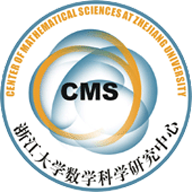(13007) PARTIAL GENERALIZATIONS OF SOME CONJECTURES IN LOCALLY SYMMETRIC LORENTZ SPACES

Abstract. In this paper, first we give a notion for linear Weingarten spacelike hypersurfaces with P + aH = b in a locally symmetric Lorentz space Ln+11 . Furthermore, we study complete or compact linear Weingarten spacelike hypersurfaces in locally symmetric Lorentz spaces Ln+1 1 satisfying some curvature conditions. By modifying Cheng-Yau’s operator  given in , we introduce a modified operator L and give new estimates of L(nH) and (nH) of such spacelike hypersurfaces. Finally, we give partial generalizations of some Conjectures in locally symmetric Lorentz spaces Ln+11 .

• Partial generalizations of some Conjectures in locally symmetric Lorentz spaces.pdf